IMO Shortlist 2014 problem G2

Kvaliteta:
Avg: 0,0
Težina:
Avg: 6,0

Let$ABC$ be a triangle. The points$K$,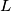$L$, and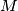$M$ lie on the segments$BC$,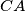$CA$, and$AB$, respectively, such that the lines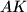$AK$,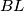$BL$, and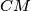$CM$ intersect in a common point. Prove that it is possible to choose two of the triangles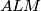$ALM$,$BMK$, and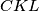$CKL$ whose inradii sum up to at least the inradius of the triangle$ABC$.

(Estonia)

Izvor: https://www.imo-official.org/problems/IMO2014SL.pdf